﻿ 仓储作业中多搬运机器人动态路径优化 Dynamic Path Planning for Multi-Robot in Warehousing Operations

Artificial Intelligence and Robotics Research
Vol. 08  No. 02 ( 2019 ), Article ID: 30316 , 15 pages
10.12677/AIRR.2019.82009

Dynamic Path Planning for Multi-Robot in Warehousing Operations

Xinmiao Wang

School of Economics and Management, Beijing Jiaotong University, Beijing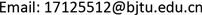Received: Apr. 26th, 2019; accepted: May 13th, 2019; published: May 21st, 2019ABSTRACT

Handling robots have been widely used in the picking system of “part-to-picker” in un-manned warehouse. However, there are still problems such as unreasonable path seeking planning and path conflict in the collaborative operation of multi-handling robot. Considering the load balance of the path and the turning time of the handling robot, the path planning model of the multi-handling robot with time window is established. Combined with the obstacle avoidance strategy of adjusting the priority, an improved “offline-online” two-stage dynamic path planning algorithm was proposed to realize the combination of global off-line path planning and online conflict avoidance, thus improving the operation efficiency of the un-manned warehouse system. Finally, three sets of simulation experiments are designed to verify the effectiveness of the improved algorithm by comparing the total running time of the handling robots.

Keywords:Multi-Handling Robot, Path Planning, Time Window, Two-Stage, Dynamic1. 引言

“货到人”拣选系统的优化对提升电商企业的市场竞争力具有重要作用  。部分研究者对智能仓库系统中的一些简化子问题开展了初步的研究工作，包括Hazard  等学者研究静态货位分配问题、Li  等研究静态任务指派和路径规划问题等。但这些研究工作都是针对静态情况的，并没有考虑实际订单拣选过程的连续性和各个环节之间的关联性。研究结果还无法解决“货到人”拣选系统中的实际问题，因此必须研究动态的路径规划策略  。

2. 带有时间窗的多搬运机器人路径规划模型

2.1. 问题描述

1) 为搬运机器人分配任务。由于一个搬运机器人不能同时执行多项订单任务，因此将任务分配给距离货架最近的空闲搬运机器人，为其规划自起点至终点的最优行走路径。并根据任务下达的时间为机器人设置优先级，任务下达越早，机器人的优先级越高；

2) 领取任务后的搬运机器人由空闲变为工作状态，自所在位置按照系统规划好的路径行驶至任务货架处，将任务货架顶起，机器人由空载变为负载状态；

3) 带有任务货架的搬运机器人行驶至目标拣选台，等待拣选。当前拣选任务完成后检查是否存在其他拣选任务，若有，移动至指定拣选台等待拣选；否则，根据系统给出的目标位置，为搬运机器人设计回程最优路径，将任务货架运回。搬运机器人此时的状态变为空载、空闲；

4) 判断搬运机器人是否需要充电，如需充电，行驶至充电桩处进行充电，否则释放搬运机器人；

5) 转至步骤1)，重复执行以上操作，直至无拣选任务。

1) 每条边上运行的搬运机器人数量不能超过其能承载的最大数量；

2) 每个搬运机器人在行驶过程中按匀速行驶，机器人遇到障碍时的停车减速时间忽略不计；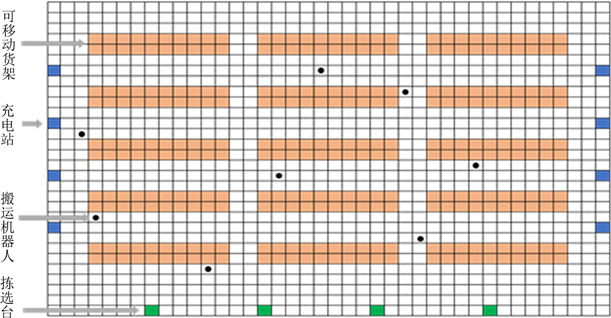Figure 1. Environment map of warehouse operation

3) 同向行驶时，为防止前面的机器人因突然停止(故障)导致两车相撞，规定机器人在行驶过程中需与前车保持安全距离。本文将机器人的安全车距定为，即车身长度 + t秒内车辆的行驶长度；

4) 搬运机器人在运行过程中遇到的随机动态障碍物(人员进入)由搬运机器人的车载传感器和定位系统解决，模型及算法只面向仓库中的货架障碍以及运行期间搬运机器人之间的冲突。

2.2. 符号说明

1) 相关参数

$i,j,k$ ：路径节点， $i,j,k\in V$

${w}_{jk}$ ：边的权重

${m}_{b}$ ：运输任务标识， $b=1,2,\cdots ,p$

${h}_{a}$ ：搬运机器人标识， $a=1,2,\cdots ,q$

${t}_{jk}^{m}$ ：搬运机器人在路径 ${e}_{jk}$ 上的时间窗

${t}_{{h}_{a}}^{jk}$ ：车辆经过路径 ${e}_{jk}$ 的时间

${N}_{kj}^{{t}_{jk}^{m}}$ ：路径 ${e}_{jk}$ 上， ${t}_{jk}^{m}$ 时间窗内节点k向节点j方向运行的搬运机器人数量

${N}_{jk}^{{t}_{jk}^{m}}$ ：路径 ${e}_{jk}$ 上， ${t}_{jk}^{m}$ 时间窗内节点j向节点k方向运行的搬运机器人数量

${e}_{jk}=\left\{\begin{array}{l}1,\text{\hspace{0.17em}}\text{\hspace{0.17em}}节点\text{ }j\text{ }到节点\text{ }k\text{ }之间的路径存在\\ 0,\text{\hspace{0.17em}}\text{\hspace{0.17em}}其他\end{array}$

${x}_{{h}_{a}}^{{t}_{jk}^{m}}=\left\{\begin{array}{l}1,\text{\hspace{0.17em}}\text{\hspace{0.17em}}\text{\hspace{0.17em}}由节点\text{ }j\text{ }向\text{ }k\text{ }行驶的搬运机器人在时间窗{t}_{jk}^{m}内到达\\ 0,\text{\hspace{0.17em}}\text{\hspace{0.17em}}其他\end{array}$

${z}_{{h}_{a}}^{{t}_{jk}^{m}}=\left\{\begin{array}{l}1,\text{\hspace{0.17em}}\text{\hspace{0.17em}}\text{\hspace{0.17em}}由节点\text{ }k\text{ }向\text{ }j\text{ }行驶的搬运机器人在时间窗{t}_{jk}^{m}内到达\\ 0,\text{\hspace{0.17em}}\text{\hspace{0.17em}}其他\end{array}$

2) 决策变量

${t}_{jk}^{s}$ ：经过节点 $j,k$ 上的直线路段所耗费的时间

${t}_{jk}^{c}$ ：经过节点 $j,k$ 上的拐弯路段所耗费的时间

${t}_{w}$ ：搬运机器人 ${h}_{a}$ 的耗费的额外等待时间。

2.3. 模型建立

2.3.1. 时间窗

${t}_{jk}^{a}=\left[{t}_{jk}^{ai},{t}_{jk}^{ao}\right]$ (1)

q个搬运机器人在边 ${e}_{jk}$ 上时间窗集合为：

${t}_{jk}^{m}=\left\{{t}_{jk}^{1},{t}_{jk}^{2},\cdots ,{t}_{jk}^{q}\right\}$ (2)

2.3.2. 带有时间窗的多搬运机器人路径规划模型

$\mathrm{min}Z=\underset{a=1}{\overset{p}{\sum }}{h}_{a}\left({t}_{ji}^{s}\ast {w}_{ji}+{t}_{ji}^{c}+{t}_{ik}^{s}\ast {w}_{ik}+{t}_{w}\right)$ (3)

s.t.

$\underset{a=1}{\overset{p}{\sum }}{h}_{a}\le p$ (4)

$\underset{a=1}{\overset{p}{\sum }}\underset{b=1}{\overset{q}{\sum }}{x}_{ab}=q$ (5)

$\underset{a=1}{\overset{p}{\sum }}{x}_{ab}=1$ (6)

${t}_{jk}^{m}=\left\{\left[{t}_{jk}^{ai},{t}_{jk}^{ao}\right]\right\}$ (7)

${N}_{jk}^{{t}_{jk}^{m}}={e}_{jk}\ast \underset{i=1}{\overset{q}{\sum }}{x}_{{h}_{a}}^{{t}_{jk}^{m}}$ (8)

${N}_{kj}^{{t}_{jk}^{m}}={e}_{jk}\ast \underset{i=1}{\overset{q}{\sum }}{z}_{{h}_{a}}^{{t}_{jk}^{m}}$ (9)

${N}_{kj}^{{t}_{jk}^{m}}+{N}_{kj}^{{t}_{jk}^{m}}\le \frac{{L}_{jk}}{{L}_{v}}$ (10)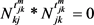(11)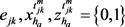(12)

3. 带有时间窗的多搬运机器人两阶段动态路径规划算法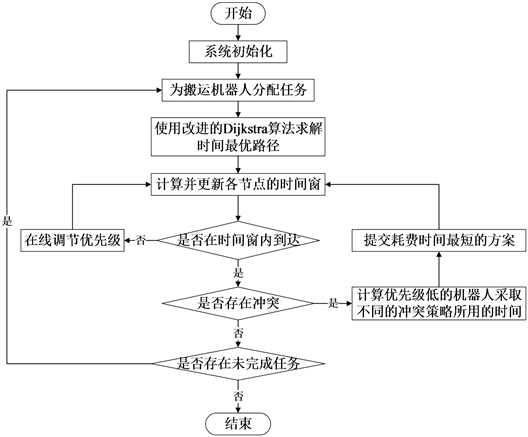Figure 2. Flowchart of multi-handling robot path planning algorithm

3.1. 离线阶段–带有路径热度的Dijkstra算法求解最优路径

1) 利用传统的Dijkstra算法为各个搬运机器人从任务起始点到终点的路径进行初步规划；

2) 对1)中的路径进行分解，统计时间周期 $\delta$ 内各个路段的利用频次 ${h}_{k}$

3) 当搬运机器人通过当前路径时， ${b}_{g}$ 自动减1；

4) 构造路径繁忙程度对Dijkstra算法选择路径的影响系数函数：

$F\left(fb\right)=\left({b}_{g}+1\right){h}_{k}/\underset{g=1}{\overset{p}{\sum }}\underset{k=1}{\overset{E}{\sum }}{b}_{g}{h}_{k}$ (13)

${w}_{jk}=1+F\left(fb\right)$ (14)

5) 利用Dijkstra算法，根据加权后的路径长度对搬运机器人的路径进行规划，直至完成所有的任务。

3.2. 在线阶段–基于调节优先级的在线交通控制策略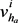v点是搬运机器人  经过的第i个节点

${v}_{{h}_{a}}^{j}$ a车是经过节点v的第j辆搬运机器人

$A=\left\{{v}_{{h}_{a}}^{1},{v}_{{h}_{a}}^{2},\cdots ,{v}_{{h}_{a}}^{n}\right\}$ ，表示搬运机器人所经过的节点，  ，表示经过某节点的搬运机器人车辆，数组A、B中的节点在搬运机器人经过某一节点后更新。

1) 车辆 ${h}_{a}$ 行驶至节点v，检测v是否为的第i个节点， ${h}_{a}$ 是否为通过此节点的第j辆车；

2) 若节点v未被其他搬运机器人占用， ${h}_{a}$ 是第j个通过该节点的搬运机器人，即在该点的优先级最高，则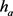继续行驶，通过节点v；

3) 若节点v未被其他搬运机器人占用， ${h}_{a}$ 不是第j个通过该节点的搬运机器人，即前一辆车 ${h}_{a-1}$ 未通过此节点，需判断搬运机器人 ${h}_{a-1}$ 所处的位置是否处于经过节点v的下一行驶路段范围外。若是，执行步骤①；若否，执行步骤②：

①调整节点v上 ${h}_{a}$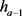的优先级，改变车辆的通过顺序；

②停车等待，设定等待时长为 ${T}_{w1}$ ，若在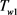范围内通过节点v，则 ${h}_{a}$ 继续按照原始路径行走，通过节点v；若在 ${T}_{w1}$ 范围内  未通过节点v，则需重新规划至终点的路径。若路径存在，则将其更改为  的行驶路径，若不存在，则继续停车等待处理；

4) 若节点v被其他搬运机器人占用，则停车等待，设定等待时长为 ${T}_{w2}$ 。若等待时长超出 ${T}_{w2}$ ，节点v仍处于被占用状态，则考虑重新规划路径，若不存在其他可行路径，也就是说v是搬运机器人到达终点的必经节点，则停车，状态显示为故障状态。

3.3. 在线冲突规避

①若两辆相向行驶的搬运机器人均按原始路径匀速行驶时，存在着相向冲突，那么判断两个搬运机器人的优先级。优先级高的搬运机器人正常通过，另一辆车则进行在线调节，判断等待、二次规划路径所需的时间，选择耗时较少的策略。

②若两辆相向行驶的搬运机器人中存在着一辆搬运机器人在停车等待，存在着相向冲突，那么判断两个搬运机器人的优先级。若暂停的搬运机器人优先级最高，那么继续等待，另一搬运机器人进行在线调节，判断等待、二次规划路径所需的时间，选择耗时较少的策略；若行驶的搬运机器人优先级最高，则搬运机器人正常通过，暂停等待的搬运机器人行驶至下一空闲节点执行等待命令。

③若两辆相向行驶的搬运机器人中存在着一辆搬运机器人在执行拣选操作，存在着相向冲突，那么搬运机器人继续执行拣选工作，另一搬运机器人选择进行在线调节策略。在冲突解决策略中，执行拣选工作的搬运机器人优先级最高。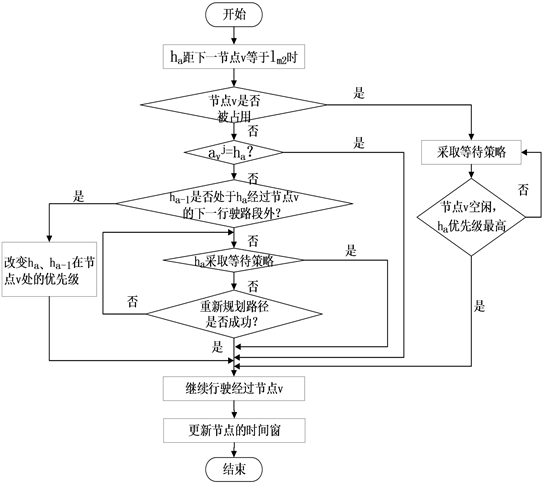Figure 3. The flow chart of online adjust the priority strategy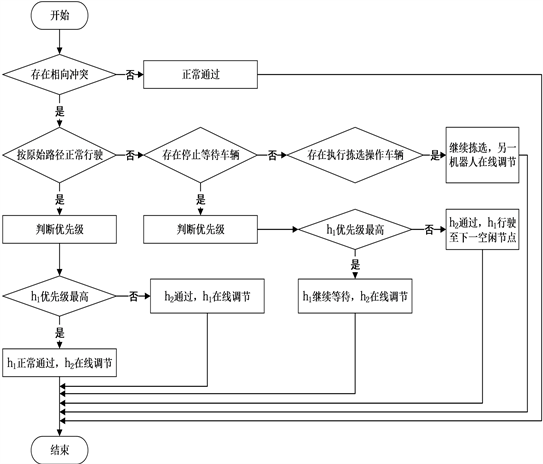Figure 4. Strategy for resolving conflicts in the opposite direction

4. 仿真实例

4.1. 第一组对比实验Table 1. Task parameter settings of the handling robot system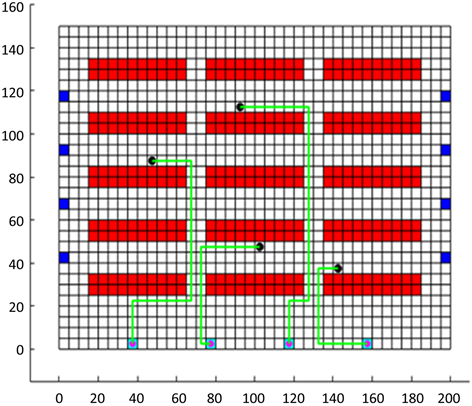Figure 5. Traditional Dijkstra algorithm for path planning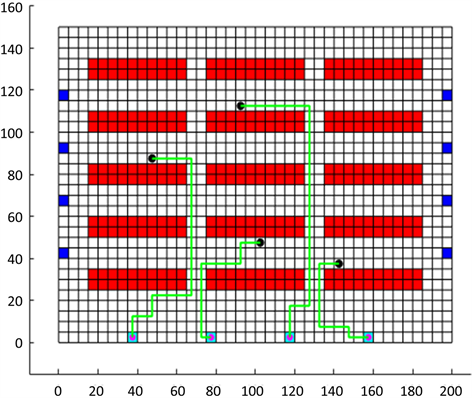Figure 6. Improved Dijkstra algorithm for path planning

4个任务的总运行时间和总运行距离对比见图7。实验结果表明，若综合考虑各条路径的热度以转向所需时间后，使用改进的Dijkstra算法求解的路径，搬运机器人的总运行距离比传统算法节约了14.45米；总运行时间节省了8.45秒，搬运机器人的效率与传统算法相比有明显的提升，体现了改进算法的有效性。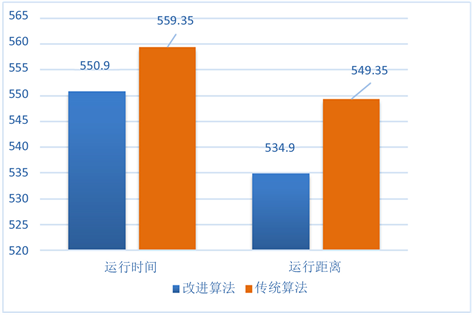Figure 7. Comparison of results before and after algorithm improvement

4.2. 第二组对比实验Table 2. Task parameter settings of the handling robot systemTable 3. Path planning results based on traditional Dijkstra algorithm under different example sizesTable 4. Path planning results based on improved Dijkstra algorithm under different example sizes

4.3. 第三组对比实验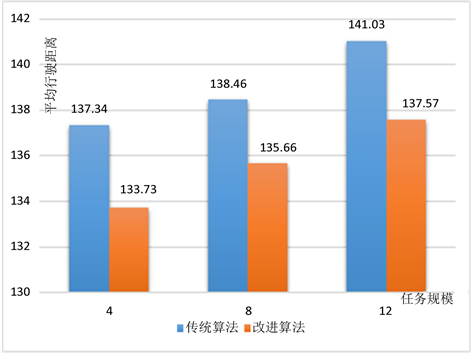Figure 8. Average distance comparison of the handling robots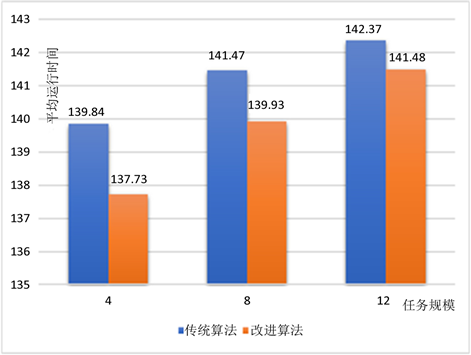Figure 9. Average running time comparison of the handling robotsTable 5. Task parameter settings of the handling robotsFigure 10. Simulation path diagram of handling robotsTable 6. Simulation results of handling robot under different strategies

5. 结论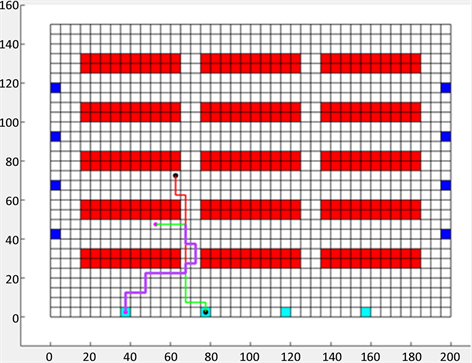Figure 11. Path diagram for online adjustment strategy

Dynamic Path Planning for Multi-Robot in Warehousing Operations[J]. 人工智能与机器人研究, 2019, 08(02): 68-82. https://doi.org/10.12677/AIRR.2019.82009

1. 1. 胡国盛. 我国电商企业物流体系优化分析[J]. 商业经济研究, 2017(19): 106-108.

2. 2. Hazard, C.J. and Wurman, P.R. (2006) Alphabet Soup: A Testbed for Studying Resource Allocation in Multi-Vehicle Systems. Proceedings of the 2006 AAAI Workshop on Auction Mechanisms for Robot Coordination, Boston, 17 July 2006, 23-30.

3. 3. Li, W., Jiang, L. and Li, Z. (2016) Research on the Task Assignment Problem of Warehouse Robots in the Smart Warehouse. 12th International Symposium on Operations Research and Its Applications in Engineering, Technology and Management, Luoyang, 21-24 August 2015, 29-33.

4. 4. Yeung, W.-K., Choi, T.M. and Cheng, T.C.E. (2011) Supply Chain Scheduling and Coordination with Dual Delivery Modes and Inventory Storage Cost. International Journal of Production Economics, 132, 223-229. https://doi.org/10.1016/j.ijpe.2011.04.012

5. 5. Khorshid, M.M., Holte, R.C. and Sturtevant, N.R. (2013) A Polynomial-Time Algorithm for Non-Optimal Multi-Agent Pathfinding. Symposium on Combinatorial Search. Proceedings of the 4th Annual Symposium on Combinatorial Search, Castell de Cardona, Barcelona, 15-16 July 2011, 76-83.

6. 6. Chang, W.K. and Tanchoco, J.M.A. (1991) Journal of Production Research Conflict-Free Shortest Time Bidirectional AGV Routing. International Journal of Production Research, 29, 2377-2391. https://doi.org/10.1080/00207549108948090

7. 7. Ma, Y., Wang, H., Xie, Y. and Guo, M. (2014) Path Planning for Multiple Mobile Robots under Double-Warehouse. Information Sciences, 278, 357-379. https://doi.org/10.1016/j.ins.2014.03.058

8. 8. Umar, U.A., Ariffin, M.K.A. and Ismail, N. (2012) Priority-Based Genetic Al-gorithm for Conflict-Free Automated Guided Vehicle Routing. Journal of Engineering Valuation and Cost Analysis, 50, 732-739.

9. 9. Desrochers, M., Desrosiers, J. and Solomon, M. (1992) A New Optimization Algorithm for the Vehicle Routing Problem with Time windows. Operation Research, 40, 199-415. https://doi.org/10.1287/opre.40.2.342

10. 10. Wang, W. and Goh, W.B. (2015) A Stochastic Algorithm for Makespan Minimized Multi-Agent Path Planning in Discrete Space. Applied Soft Computing, 30, 287-304. https://doi.org/10.1016/j.asoc.2015.01.046

11. 11. Hidalgo-Paniagua, A., Miguel Vega-Rodríguez, A., Ferruz, J. and Pavón, N. (2015) MOSFLA-MRPP: Multi-Objective Shuffled Frog-Leaping Algorithm Applied to Mobile Robot Path Planning. Engineering Applications of Artificial Intelligence, 44, 123-136. https://doi.org/10.1016/j.engappai.2015.05.011

12. 12. Ma, H., Koenig, S., Ayanian, N., et al. (2017) Overview: Generalizations of Multi-Agent Path Finding to Real-World Scenarios. Computer Science, arXiv: 1702.05515.

13. 13. Miao, H. and Tian, Y.C. (2013) Dynamic Robot Path Planning Using an Enhanced Simulated Annealing Approach. Applied Mathematics and Computation, 222, 420-437. https://doi.org/10.1016/j.amc.2013.07.022

14. 14. Standley, T.S. and Korf, R.E. (2011) Complete Algorithms for Cooperative Pathfinding Problems. International Joint Conference on Artificial Intelligence. Proceedings of the 22nd International Joint Conference on Artificial Intelligence, Barcelona, 16-22 July 2011, 223-229.

15. 15. Sharon, G., Stern, R., Felner, A. and Sturtevant, N.R. (2015) Conflict-Based Search for Optimal Multi-Agent Pathfinding. Artificial Intelligence, 219, 40-66. https://doi.org/10.1016/j.artint.2014.11.006

16. 16. 何小锋, 马良. 带时间窗车辆路径问题的量子蚁群算法[J]. 系统工程理论与实践, 2013, 33(5): 1255-1261.

17. 17. 张立峰, 易万里, 刘晓兰. 基于两阶段算法的大规模成品油二次配送优化[J]. 系统工程理论与实践, 2016, 36(11): 2951-2963.

18. 18. Maza, S. and Castagna, P. (2005) A Performance-Based Structural Policy for Con-flict-Free Routing of Bidirectional Automated Guided Vehicles. Computers in Industry, 56, 719-733. https://doi.org/10.1016/j.compind.2005.03.003

19. 19. Lee, J.H., Lee, B.H. and Choi, M.H. (1998) A Real-Time Traffic Control Scheme of Multiple AGV Systems for Free Minimum Time Motion: A Routing Table Approach. IEEE Transactions on Systems, Man, and Cybernetics—Part A: Systems and Humans, 28, 347-358. https://doi.org/10.1109/3468.668966

20. 20. 孟祥虎, 胡蓉, 钱斌. 求解带时间窗车辆路径问题的有效混合PBIL算法[J]. 系统工程理论与实践, 2014, 34(10): 2701-2709.